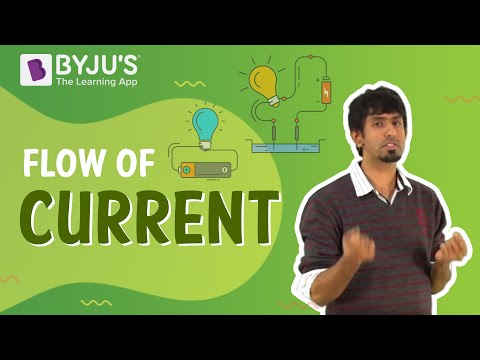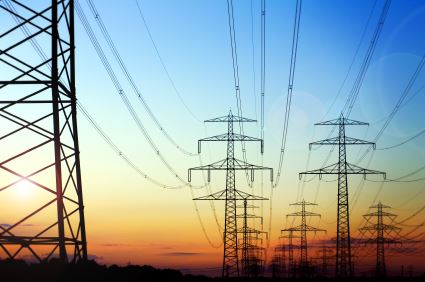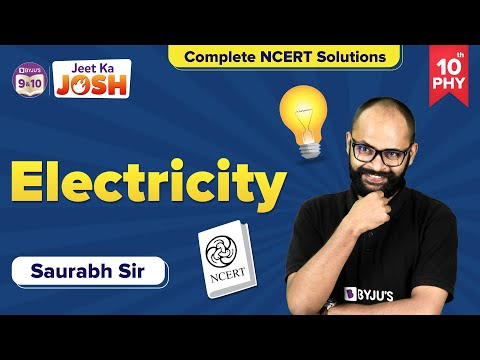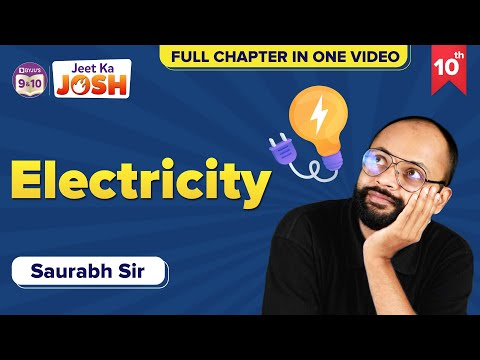# Electric Power

In physics, electric power measures the rate of electrical energy transfer by an electric circuit per unit of time. Denoted by P and measured using the SI unit of power which is watt or one joule per second. Electric power is commonly supplied by electric batteries and produced by electric generators.

## Electric Power Definition

Electric power is the rate at which work is done or energy is transformed into an electrical circuit. Simply put, it is a measure of how much energy is used in a span of time.

 Symbol P SI Unit Watt, joule per second Scalar or Vector Scalar Quantity Formula P = VI

## Why shouldn’t you touch electrical equipment with wet hands? Find out answer to this question by watching the video.## Electric Power Formula

The formula for electric power is given by

 P = VI

where,

P is the power

V is the potential difference in the circuit.

I is the electric current.

Power can also be written as

 P = I2R P = V2/ R

The above two expressions are obtained by using Ohm’s law, Where, voltage, current, and resistance are related by the following relation:

V = IR
Where,

• R is the resistance in the circuit.
• V is the potential difference in the circuit
• I is the electric current### Power-Energy Formula

Since power is the rate of energy consumption, energy can directly be calculated using

 $$\begin{array}{l}P=\frac{E}{t}\end{array}$$

Where,

E is the energy consumption (in Joules)

t is the time in seconds

 $$\begin{array}{l}P=\frac{VQ}{t}\end{array}$$

Where,

• Q is the charge (in Coulombs)
• V is potential difference in volts
• t is the time in seconds

## Electric Power Problems

Based on the information given above, try the application problem given below:

Example:

A 9V battery is connected to a resistor having a resistance of 10 Ω. What is the current and power across the resistor?

Solution:

I = V/R = 9/10 = 0.9 A

P = VI = 9 × 0.9 = 8.1 J/s or 8.1 W

## Watch the video and solve complete NCERT exercise questions in the chapter Electricity Class 10## Frequently Asked Questions – FAQs

Q1

### Define electric Power.

Electric power is the rate at which work is done or energy is transferred in an electrical circuit. Simply put, it is a measure of how much energy is used in a span of time.
Q2

### Write the SI unit of electric power

The SI unit of electric power is watt.
Q3

### Write the electric power formula and explain the terms.

The electric power is given by P = VI, where V is the potential difference, I is the electric current and P is the electric power.
Q4

### Is electric power a scalar quantity or vector quantity?

Electric power is scalar quantity.
Q5

### How can we express power using Ohm’s law?

The power formula can be rewritten using Ohm’s law as P =I2R or P = V2/R, where V is the potential difference, I is the electric current, R is the resistance, and P is the electric power.

## Watch the video and solve important questions in the chapter Electricity Class 10Stay tuned with BYJU’S for more such interesting articles. Also, register to “BYJU’S-The Learning App” for loads of interactive, engaging physics-related videos and total academic assistance.

Test Your Knowledge On Electric Power!